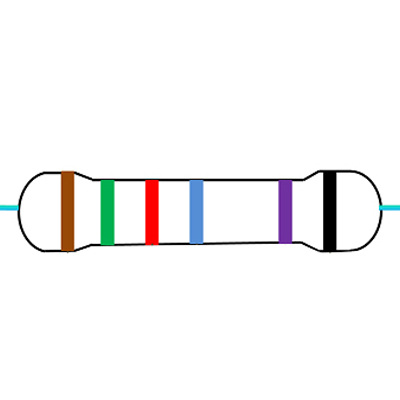# 6 Band Resistor Color Code Calculator

## 6 Band Resistor Color Code Calculator Tool

This tool is used to calculate the information for color banded axial lead resistors of 6 band. Select the number of bands, then their colors to determine the value and tolerance of the resistors.## 6 Band Resistor

Resistors with 6 bands are usually for high precision resistors that have an additional band to specify the temperature coefficient (ppm/˚C = ppm/K). The most common color for the sixth band is brown (100 ppm/˚C). This means that for a temperature change of 10 ˚C, the resistance value can change 1000 ppm = 0.1%.

### How to read a typical 6-band resistor

The first three color bands represent significant digits, the fourth band represents the decimal multiplier, the fifth band represents the tolerance, and the sixth band represents the temperature coefficient.